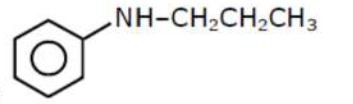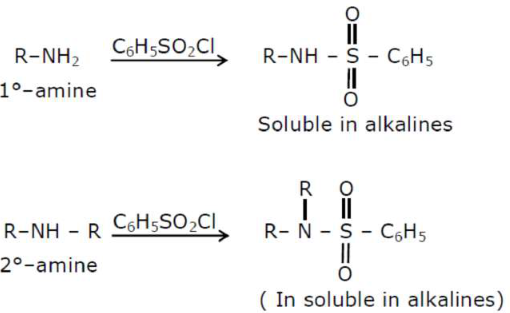# An amine on reaction with benzenesulphonyl chloride produces a compound insoluble in alkaline solution.Question:

An amine on reaction with benzenesulphonyl chloride produces a compound insoluble in alkaline solution. This amine can be prepared by ammonolysis of ethyl chloride. The correct structure of amine is:

1. $\mathrm{CH}_{3} \mathrm{CH}_{2} \mathrm{CH}_{2} \stackrel{\mathrm{H}}{\mathrm{N}}-\mathrm{CH}_{2} \mathrm{CH}_{3}$

2. $\mathrm{CH}_{3} \mathrm{CH}_{2} \mathrm{CH}_{2} \mathrm{NHCH}_{3}$

3.4. $\mathrm{CH}_{3} \mathrm{CH}_{2} \mathrm{NH}_{2}$

Correct Option:

Solution:According to the question the amine should be $2^{\circ}$-amine, in which one of the alkyl group should be ethyl, because it can be formed by ammonolysis of ethyl chloride